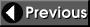#### Importance Sampling

An elegant solution to molecular Monte Carlo simulations that sample from Boltzmann distribution was provided by Metropolis and co-workers of the Los Alamos team. Instead of accumulating configurations randomly, and then evaluating their probability-weighed contribution to the desired property we can accumulate configurations according to their Boltzmann probability and then take a simple arithmetic average. This is accomplished in the Metropolis Monte Carlo algorithm by first making a random move, then evaluating the Boltzmann probability of such a move and comparing the probability against a random number. If the Boltzmann probability of the move is larger than the random number, the move is accepted; otherwise the system is returned to its original configuration. At the end, a set of configurations is obtained according to Boltzmann statistics and the expectation value of a property is obtained as a simple arithmetic average of property values from individual accepted configurations:#### Metropolis Monte Carlo algorithm

The computer implementation of importance sampling from the Boltzmann distribution is known as the Metropolis Monte Carlo algorithm. We illustrate such implementation by calculating the average position, the root mean square displacement, and the average energy of a classical particle in harmonic potential. Harmonic potential is often used in molecular simulations to describe bond vibrations. Below, a core of the Metropolis Monte Carlo algorithm is implemented in the Python programming language. The complete program can be downloaded here.

```#
# Hamiltonian: Harmonic potential
#
def hamiltonian(r):
pot = 0.5 * k * (r - r_eq)**2
return pot
#
# Monte Carlo code
#
acc = 0
mc_dst = 0.0
mc_dst2 = 0.0
mc_ener = 0.0

r = r_ini

for point in range(1,points):
r_new = r + step * ( random() - 0.5 ) * 2.0	# Move particle randomly
v     = hamiltonian(r)
v_new = hamiltonian(r_new)
if v_new < v:                                # Downhill move always accepted
r = r_new
v = v_new
acc = acc + 1
else:                                        # Uphill move accepted with luck
A_mov = exp( -beta *(v_new - v) )
if A_mov > random():
r = r_new
v = v_new
acc = acc + 1
# Update regardless of acceptance!
mc_dst  = mc_dst + r
mc_dst2 = mc_dst2 + r*r
mc_ener = mc_ener + v

```Educational material by Dr. Kalju Kahn, Department of Chemistry and Biochemistry , UC Santa Barbara. 2005